# Frank solutions for ICSE Class 10 Mathematics Part 2 chapter 14 - Symmetry [Latest edition]

#### Chapters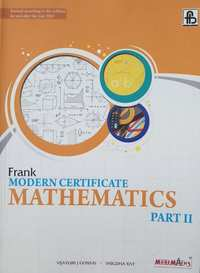## Chapter 14: Symmetry

Exercise 14.1
Exercise 14.1

### Frank solutions for ICSE Class 10 Mathematics Part 2 Chapter 14 Symmetry Exercise 14.1

Exercise 14.1 | Q 1

Construct an isosceles triangle whose equal sides are 7 cm each and the base side is 5 cm. Draw all its lines of symmetry.

Exercise 14.1 | Q 2

Take a graph paper and mark the points A(2,0), B(2,8) and C(S,4) on it. Taking AB as the line of symmetry, obtain and write the co-ordinates of point D. Complete the quadrilateral ABCD and give its geometrical name.

Exercise 14.1 | Q 3

Take a graph paper and mark the points P(2, 1), Q(7, 1) and R(7,5). Taking QR as the line of symmetry, obtain and write the co-ordinates of point S.

Exercise 14.1 | Q 4

A(8,2) and B(6,4) are the vertices of a figure which is symmetrical about x = 6 and y = 2. Complete the figure and give the geometrical name of the figure.

Exercise 14.1 | Q 5

A(2,2) and B(5,5) are the vertices of a figure which is symmetrical about xaxis. Complete the figure and give its geometrical name.

Exercise 14.1 | Q 6

A( 4, 1 ), B(2,3) and C( 5,6) are the vertices of a figure which is symmetrical about x=7. Complete the figure and give the geometrical name of the figure if any.

Exercise 14.1 | Q 7.1

In the following figure, the line of symmetry has been drawn with a dotted line. Identify the corresponding sides and the corresponding angles about the line of symmetry.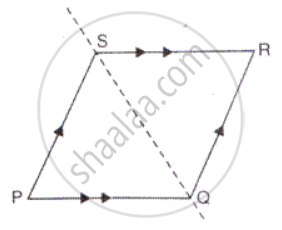Exercise 14.1 | Q 7.2

In the following figures, the line of symmetry has been drawn with a dotted line. Identify the corresponding sides and the corresponding angles about the line of symmetry.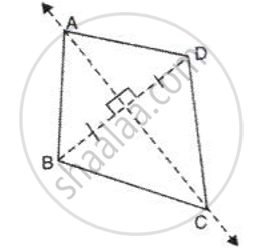Exercise 14.1 | Q 7.3

In the following figures, the line of symmetry has been drawn with a dotted line. Identify the corresponding sides and the corresponding angles about the line of symmetry.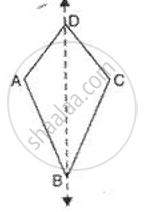Exercise 14.1 | Q 7.4

In the following figures, the line of symmetry has been drawn with a dotted line. Identify the corresponding sides and the corresponding angles about the line of symmetry.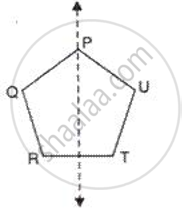Exercise 14.1 | Q 8.1

Draw the line of symmetry of the following figure and also mark their points of symmetry, if any: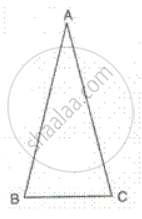Exercise 14.1 | Q 8.2

Draw the line of symmetry of the following figure and also mark their points of symmetry, if any: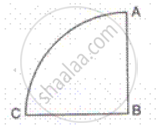Exercise 14.1 | Q 8.3

Draw the line of symmetry of the following figure and also mark their points of symmetry, if any: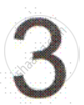Exercise 14.1 | Q 8.4

Draw the line of symmetry of the following figure and also mark their points of symmetry, if any: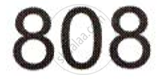Exercise 14.1 | Q 9.01

Complete the following figure, about the given lines of symmetry which are shown by dotted lines and name the figure thus obtained: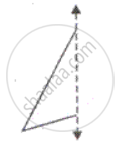Exercise 14.1 | Q 9.02

Complete the following figure, about the given lines of symmetry which are shown by dotted lines and name the figure thus obtained: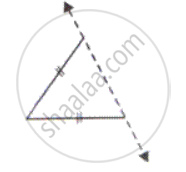Exercise 14.1 | Q 9.03

Complete the following figure, about the given lines of symmetry which are shown by dotted lines and name the figure thus obtained: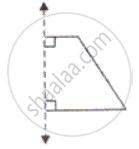Exercise 14.1 | Q 9.04

Complete the following figure, about the given lines of symmetry which are shown by dotted lines and name the figure thus obtained: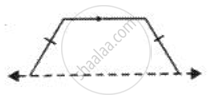Exercise 14.1 | Q 9.05

Complete the following figure, about the given lines of symmetry which are shown by dotted lines and name the figure thus obtained: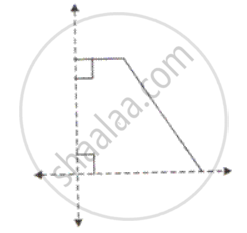Exercise 14.1 | Q 9.06

Complete the following figure, about the given lines of symmetry which are shown by dotted lines and name the figure thus obtained: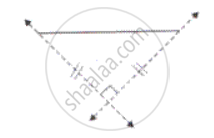Exercise 14.1 | Q 9.07

Complete the following figure, about the given lines of symmetry which are shown by dotted lines and name the figure thus obtained: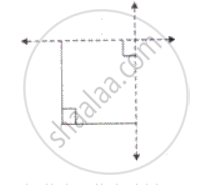Exercise 14.1 | Q 9.08

Complete the following figure, about the given lines of symmetry which are shown by dotted lines and name the figure thus obtained: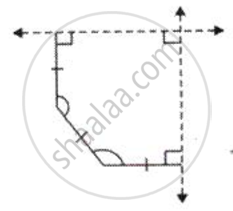Exercise 14.1 | Q 9.09

Complete the following figure, about the given lines of symmetry which are shown by dotted lines and name the figure thus obtained: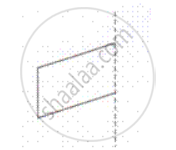Exercise 14.1 | Q 9.1

Complete the following figure, about the given lines of symmetry which are shown by dotted lines and name the figure thus obtained: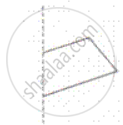Exercise 14.1 | Q 9.11

Complete the following figure, about the given lines of symmetry which are shown by dotted lines and name the figure thus obtained: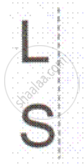Exercise 14.1 | Q 9.12

Complete the following figure, about the given lines of symmetry which are shown by dotted lines and name the figure thus obtained: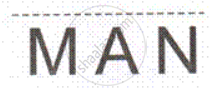Exercise 14.1 | Q 10.1

Given below are part of a geometrical figure. Complete the figure so that both axes are lines of symmetry.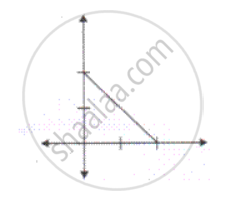Exercise 14.1 | Q 10.2

Given below are part of a geometrical figure. Complete the figure so that both axes are lines of symmetry.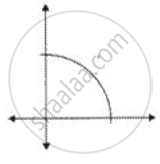Exercise 14.1 | Q 10.3

Given below are part of a geometrical figure. Complete the figure so that both axes are lines of symmetry.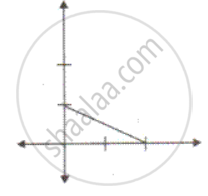Exercise 14.1 | Q 10.4

Given below are part of a geometrical figure. Complete the figure so that both axes are lines of symmetry.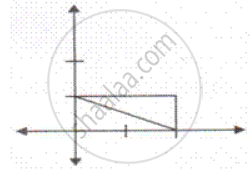Exercise 14.1 | Q 11

Construct a triangle ABC in which each side measures 5.8 cm. Draw all the possible lines of symmetry.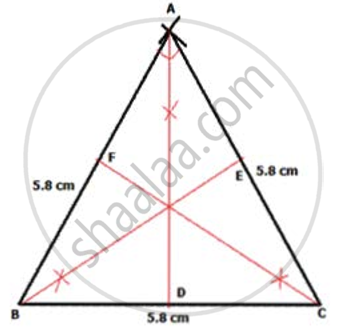Exercise 14.1 | Q 12

Construct a paralIelogra m PQRS in wh i di QR = 5. 4 cm , SR = 6. 0 cm and ∠ Q= 60°. Draw its lines of symmetry, if possible.

Exercise 14.1 | Q 13

Construct a square of side 4.8 cm and draw all its lines of symmetry .

Exercise 14.1 | Q 14

Construct a regular hexagon of side = 3.8 cm and draw all its lines of symmetry .

Exercise 14.1 | Q 15

Construct a rhombus ABCD with AB= 5.0 cm and AC= 8 cm. Draw its lines of symmetry.

Exercise 14.1 | Q 16

Construct an isosceles right-angled triangle, having hypotenuse= 8 cm. Draw its lines of symmetry.

Exercise 14.1 | Q 17

Construct a Δ ABC in whidi BA= BC= 6 cm and AC= 4.5 cm. Taking AC as line of symmetry, obtain a point D to form a quadrilateral ABCD. Name the figure ABCD.

Exercise 14.1 | Q 18

Construct a Δ PQR in which ∠ R = 90 ° ,  PQ = 5.2 cm and QR= 2.6 cm. Complete the figure taking PR as the line of symmetry and name the figure.

## Chapter 14: Symmetry

Exercise 14.1## Frank solutions for ICSE Class 10 Mathematics Part 2 chapter 14 - Symmetry

Frank solutions for ICSE Class 10 Mathematics Part 2 chapter 14 (Symmetry) include all questions with solution and detail explanation. This will clear students doubts about any question and improve application skills while preparing for board exams. The detailed, step-by-step solutions will help you understand the concepts better and clear your confusions, if any. Shaalaa.com has the CISCE ICSE Class 10 Mathematics Part 2 solutions in a manner that help students grasp basic concepts better and faster.

Further, we at Shaalaa.com provide such solutions so that students can prepare for written exams. Frank textbook solutions can be a core help for self-study and acts as a perfect self-help guidance for students.

Concepts covered in ICSE Class 10 Mathematics Part 2 chapter 14 Symmetry are Concept of Lines Symmetry, Concept of Symmetry.

Using Frank Class 10 solutions Symmetry exercise by students are an easy way to prepare for the exams, as they involve solutions arranged chapter-wise also page wise. The questions involved in Frank Solutions are important questions that can be asked in the final exam. Maximum students of CISCE Class 10 prefer Frank Textbook Solutions to score more in exam.

Get the free view of chapter 14 Symmetry Class 10 extra questions for ICSE Class 10 Mathematics Part 2 and can use Shaalaa.com to keep it handy for your exam preparation